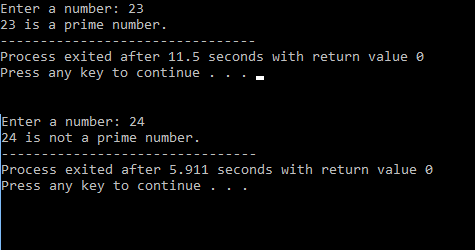# C Program to find whether a number is prime number or not.

Hey there!

Welcome to ClearUrDoubt.com.

In this post, we will look at a C program to find whether a given number is a prime number or not.

Prime Number: A number, which is divisible by only 1 and itself.

Eg.

1, 2, 3, 5, 7, 11 and so on are prime numbers.

To find whether a number(let’s say “num”) is prime or not, we need to check the remainder when we divide the given number with numbers from 2 to num/2.

This can be achieved using Modulus operator(“%”) in C. This operator gives the remainder of the division expression.

Eg.
10 % 3 = 1
11 % 3 = 2
12 % 3 = 0

Here is the C program:

Output:Happy Learning! 🙂